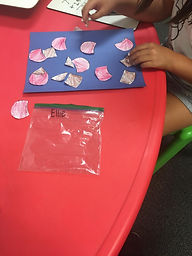## Ms. Hiral

### Target 1​

###### Lesson Type:

Review

Number Operation

:

Computation

Subtract within 1,000 (regrouping limited to only one place-value).

###### 1:

Understand why the standard algorithm for subtraction is based on place-value.

###### 2:

Understand that when subtracting a multi-digit number, each place-value is being subtracted individually to create the new number (ones are subtracted from ones, tens are subtracted from tens, etc.)

###### 3:

Understand that when adding a multi-digit number, to solve start in the lowest place-value and go left.

###### 4:

Understand how to regroup based on place-value understandings (i.e., ten ones can be composed into one ten – ten tens can be composed into one hundred).

###### 5:

Understand that the same process is utilized to subtract three or more whole numbers that is used to subtract only two numbers.

2nd

###### Vocabulary:

Subtraction, Minus, Borrow, Place-Value

Activities:

Students worked on an activity/puzzle where they practice three-digit subtraction and addition. Students created flowers by cutting out petals, coloring them, and joining them together on construction paper. Each petal has an equation on it or a number written in standard form. Students solved the equations, found the answer, and glued the flower pieces together on their paper.### Home Exploration

###### Guiding Questions:## Absent Students:

### Target 2

:

###### 1:

Use a number to represent base ten block quantities.

###### 2:

Represent a multi-digit number in expanded format.

###### 3:

Represent the composition or decomposition of three-digit numbers with an addition equation (113 = 100+10+3 or 1 hundred 1 ten and 3 ones).

###### 4:

Represent the composition or decomposition of three-digit numbers with a standard expanded format equation using both multiplication and addition (384 = (3x100) +(8x10) +(4x1)).

2nd

###### Vocabulary:

Place-Value, Expanded Format, Composition, Decomposition, Regroup

Activities:

Students created a number using the thousands, tens, and one's blocks and then wrote the equation in expanded format. Students found place-values of various numbers. Students worked on decomposing three-digit numbers based on place-value.### Home Exploration

###### Guiding Questions:### Target 3

:

###### Vocabulary:

Activities:### Home Exploration